International
Tables for
Crystallography
Volume B
Reciprocal space
Edited by U. Shmueli

International Tables for Crystallography (2006). Vol. B. ch. 2.5, p. 318   | 1 | 2 |

## Section 2.5.6.5. The method of back-projection

B. K. Vainshteinc

#### 2.5.6.5. The method of back-projection

| top | pdf |

This method is also called the synthesis of projection functions. Let us consider a two-dimensional case and stretch along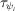each one-dimensional projection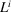(Fig. 2.5.6.5) by a certain length b; thus, we obtain the projection function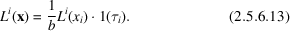Let us now superimpose h functions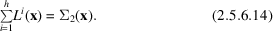The continuous sum over the angles of projection synthesis is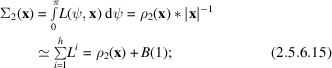this is the convolution of the initial function with a rapidly falling function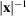(Vainshtein, 1971b). In (2.5.6.15), the approximation for a discrete set of h projections is also written. Since the functionapproaches infinity at, the convolution with it will reproduce the initial function, but with some background B decreasing around each point according to the law. At orthoaxial projection the superposition of cross sections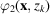arranged in a pile gives the three-dimensional structure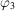.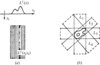Figure 2.5.6.5 | top | pdf |(a) Formation of a projection function; (b) superposition of these functions.

Radon operator. Radon (1917; see also Deans, 1983) gave the exact solution of the problem of reconstruction. However, his mathematical work was for a long time unknown to investigators engaged in reconstruction of a structure from images; only in the early 1970s did some authors obtain results analogous to Radon's (Ramachandran & Lakshminarayanan, 1971; Vainshtein & Orlov, 1972, 1974; Gilbert, 1972a).

The convolution in (2.5.6.15)may be eliminated using the Radon integral operator, which modifies projections by introducing around each point the negative values which annihilate on superposition the positive background values. The one-dimensional projection modified with the aid of the Radon operator has the form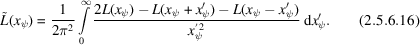Now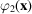is calculated analogously to (2.5.6.14), not from the initial projections L but from the modified projection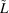: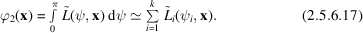The reconstruction of high-symmetry structures, in particular helical ones, by the direct method is carried out from one projection making use of its equivalence to many projections. The Radon formula in discrete form can be obtained using the double Fourier transformation and convolution (Ramachandran & Lakshminarayanan, 1971).

### ReferencesDeans, S. R. (1983). The Radon transform and some of its applications. New York: John Wiley.Google ScholarGilbert, P. F. C. (1972a). The reconstruction of a three-dimensional structure from projections and its application to electron microscopy. II. Direct methods. Proc. R. Soc. London Ser. B, 182, 89–102.Google ScholarRadon, J. (1917). Über die Bestimmung von Funktionen durch ihre Integralwerte längs gewisser Mannigfaltigkeiten. (On the determination of functions from their integrals along certain manifolds). Ber. Verh. Saechs. Akad. Wiss. Leipzig Math. Phys. Kl. 69, 262–277.Google ScholarRamachandran, G. N. & Lakshminarayanan, A. V. (1971). Three-dimensional reconstruction from radiographs and electron micrographs: application of convolutions instead of Fourier transforms. Proc. Natl Acad. Sci. USA, 68(9), 2236–2240.Google ScholarVainshtein, B. K. (1971b). Finding the structure of objects from projections. Sov. Phys. Crystallogr. 15, 781–787.Google ScholarVainshtein, B. K. & Orlov, S. S. (1972). Theory of the recovery of functions from their projections. Sov. Phys. Crystallogr. 17, 213–216.Google ScholarVainshtein, B. K. & Orlov, S. S. (1974). General theory of direct 3D reconstruction. Proceedings of International Workshop, Brookhaven National Laboratory, pp. 158–164.Google Scholar